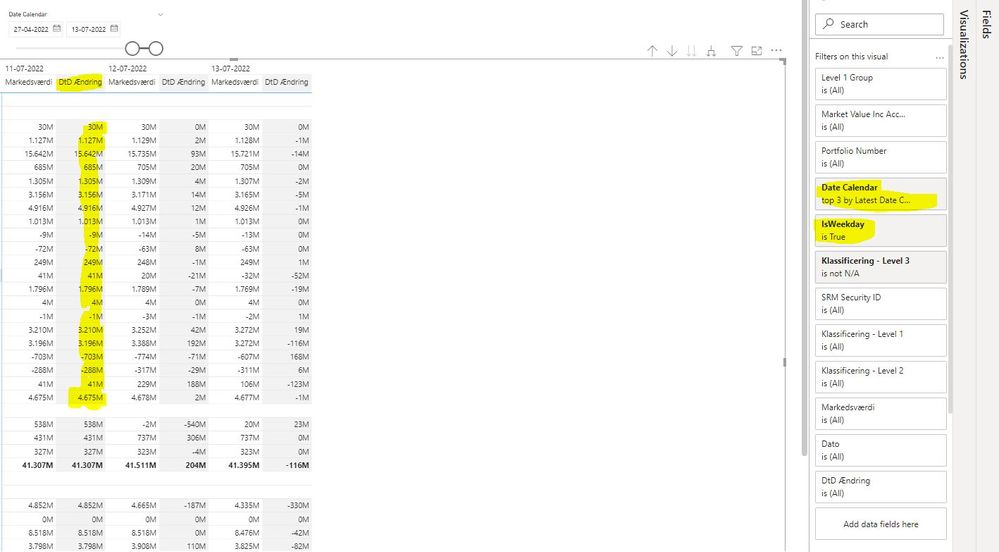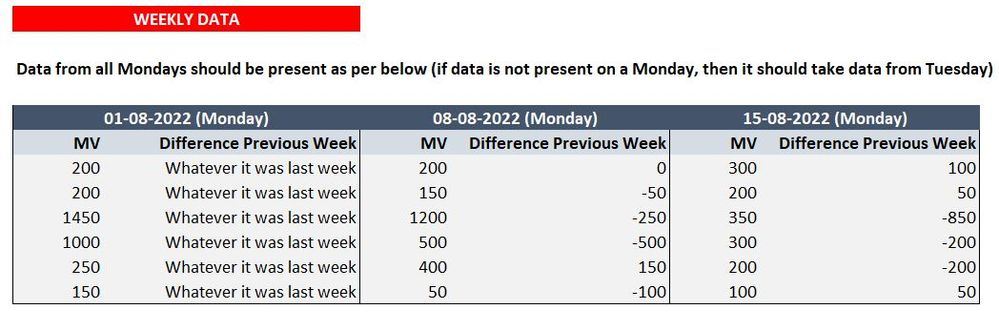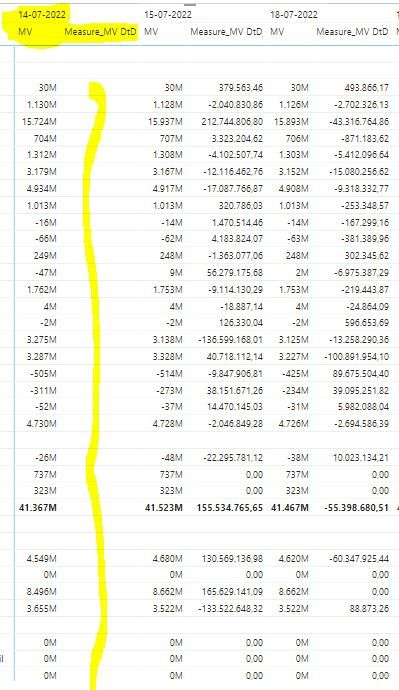cancel
Showing results for
Did you mean:Helper III

## Day to Day difference

Hi All,

I am trying to generate a day to day difference and since I only have data on weekdays and that my data lags 1 day I am facing some issues.

I have the following code:

``````Measure_DTD =
VAR vtoday = MAX('Date Table'[Date])
VAR vyesterday = IF(FORMAT(MAX('Date Table'[Date]), "DDDD")<> "Monday", MAX('Date Table'[Date])-1, MAX('Date Table'[Date])-3)
VAR vtwodays = IF(FORMAT(MAX('Date Table'[Date]), "DDDD")<> "Monday", MAX('Date Table'[Date])-2,
IF(FORMAT(MAX('Date Table'[Date]), "DDDD")<> "Tuesday", MAX('Date Table'[Date])-4,
MAX('Date Table'[Date])-5)
)
Return
IF(ISBLANK([Measure_MarketValue]),
BLANK(),
IF(FORMAT(vtoday,"DDDD") = "Monday",
CALCULATE([Measure_MarketValue],'Date Table'[Date] = vtoday) - CALCULATE([Measure_MarketValue],'Date Table'[Date] = vyesterday),

IF(FORMAT(vtoday, "DDDD") = "Tuesday",
CALCULATE([Measure_MarketValue], 'Date Table'[Date] = vtoday) - CALCULATE([Measure_MarketValue], 'Date Table'[Date] = vyesterday),
CALCULATE([Measure_MarketValue], 'Date Table'[Date] = vtoday) - CALCULATE([Measure_MarketValue], 'Date Table'[Date] = vyesterday)
)
)
)``````

Which generates the following output (Sometimes I need to show the latest 3 days with data in it and sometimes I need to show the latest 10 days i.e.)As you see the oldest date does not generate the DtD (Day to Day difference) column correctly. Does anyone know how to solve this? (I also have a column named Isweekday as a filter, which is a true/false).

Solle

1 ACCEPTED SOLUTIONCommunity Support

Hi @Solle ,

According to my knowledge, this situation may be caused by the filter "Date Calendar".

Please try to use the slicer instead of the filter and then test the following measure:

``````Measure_Previous Visible Date MV =

VAR ___currentDate = MAX( 'Date Table'[Date] )

VAR ___previousVisibleDate =

CALCULATE (

MAX ('Date Table'[Date] ),

FILTER(ALL('Date Table'),

'Date Table'[Date] < ___currentDate))

VAR ___previousVisibleDateMV =

CALCULATE ( [Measure_MarketValue],

Filter('Date Table',

'Date Table' [Date] = ___previousVisibleDate

))

RETURN

IF ( NOT ISBLANK( [Measure_MarketValue] ), ___previousVisibleDateMV )

``````
``````Measure_MV DtD =

IF(NOT ISBLANK([Measure_Previous Visible Date MV]),

[Measure_MarketValue] - [Measure_Previous Visible Date MV])``````

If this does not work, please provide me with more details about your table and your problem or share me with your pbix file after removing sensitive data.

Refer to:

How to provide sample data in the Power BI Forum

Best Regards,

Jianbo Li

If this post helps, then please consider Accept it as the solution to help the other members find it more quickly.

7 REPLIES 7Community Support

Hi @Solle ,

Could you tell me if your problem has been solved? If it is, kindly Accept it as the solution. More people will benefit from it. Or if you are still confused about it, please provide me with more details about your table and your problem or share me with your pbix file after removing sensitive data.

Refer to:

How to provide sample data in the Power BI Forum

Best Regards,

Jianbo Li

If this post helps, then please consider Accept it as the solution to help the other members find it more quickly.Community Support

Hi @Solle ,

According to my knowledge, this situation may be caused by the filter "Date Calendar".

Please try to use the slicer instead of the filter and then test the following measure:

``````Measure_Previous Visible Date MV =

VAR ___currentDate = MAX( 'Date Table'[Date] )

VAR ___previousVisibleDate =

CALCULATE (

MAX ('Date Table'[Date] ),

FILTER(ALL('Date Table'),

'Date Table'[Date] < ___currentDate))

VAR ___previousVisibleDateMV =

CALCULATE ( [Measure_MarketValue],

Filter('Date Table',

'Date Table' [Date] = ___previousVisibleDate

))

RETURN

IF ( NOT ISBLANK( [Measure_MarketValue] ), ___previousVisibleDateMV )

``````
``````Measure_MV DtD =

IF(NOT ISBLANK([Measure_Previous Visible Date MV]),

[Measure_MarketValue] - [Measure_Previous Visible Date MV])``````

If this does not work, please provide me with more details about your table and your problem or share me with your pbix file after removing sensitive data.

Refer to:

How to provide sample data in the Power BI Forum

Best Regards,

Jianbo Li

If this post helps, then please consider Accept it as the solution to help the other members find it more quickly.Helper III

Hi @v-jianboli-msft ,

Sorry for the late reply I was on holiday! But that solved my issue, appreciate your help alot thank you!

Do you know how to generate a monday to monday difference for each week (please be aware that the data is aggregated in the weekly view) So I have the total amount for the whole week on monday so it should just subtract current monday vs last week monday values (not sum the values)

I have attached an Excel printBest Regards,
SolleSuper User

Instead of doing all kinds of acrobactics in your code (which you won't understand or remember in a week's time), isn't it better to make the model easier to work with in the first place?

"I am trying to generate a day to day difference and since I only have data on weekdays and that my data lags 1 day I am facing some issues."

So, if you have data only on weekdays, extend the data so that it's also there on weekends. If your data lags 1 day, then make it so that it does not. Could that not be much easier than that? Please use Power Query to put the data in a shape that will make your journey with DAX a pleasure.Helper III

Hi @daXtreme

I just went through my DAX Calculations and tried to simplify it a bit. What I ended up with is first a measure to generate the previous day MV which is as follows:

``````Measure_Previous Visible Date MV =
VAR ___currentDate = MAX( 'Date Table'[Date] )
VAR ___previousVisibleDate =
IF (NOT ISBLANK( [Measure_MarketValue] ),
CALCULATE (
MAX ('Date Table'[Date] ),
'Date Table'[Date] < ___currentDate
)
)
VAR ___previousVisibleDateMV =
CALCULATE ( [Measure_MarketValue],
'Date Table'[Date] = ___previousVisibleDate
)
RETURN
IF ( NOT ISBLANK( [Measure_MarketValue] ), ___previousVisibleDateMV )``````

Then I created a measure to generate the difference between T and T-1 with the following code:

``````Measure_MV DtD =
IF(NOT ISBLANK([Measure_Previous Visible Date MV]),
CALCULATE([Measure_MarketValue]) - CALCULATE([Measure_Previous Visible Date MV]))``````

However this still provides me with blank at the end of the dates in place:Do you know how to solve this, with this more simpler code?

Thank you.
SolleHelper III

Hi @daXtreme

Thank you for your reply! I am sadly not in a place where I can modify my data that much, as I am requesting from a SQL Database. However I have a proper date table, which contains a lot of different information related to dates.

The reason why the data is lagging is that i.e. Today I am able to generate the market values from yesterday, since the day has to go past such that I am able to calculate prices, for the whole portfolio.

I am not a strong DAX coder and I am fairly new to PowerBI, so I am trying to learn as much as possible. You do not have any proper DAX solution to my problem, as I am not able to modify my data that much?

Thank you.
SolleSuper User

As far as I know, even data coming from SQL can be modified and you can add to it via DAX (instead of Power Query). You just have to turn your model into a composite one. Then you'll be able to mix imported and remote tables in one model. Just try to read upon "composite models" in Microsoft's documentation on Power BI.

If you have to modify a date table, it's even easier because such a table can be imported into a composite model and you can then do whatever you like with it. So, for instance, you could add a column which will have days shifted by 1 day forward or backward.

When I have a challenge that requires a lot of DAX wizardry... I always back off and think about my model. Because it's the model that you should adjust, not the code. Code should always be simple and the model should always facilitate simple calculations. Simple means understandable and fast. If you start creating complex DAX, you'll be in for a surprise sooner rather than later.

By the way, measures are always IMPLICITLY wrapped in CALCULATE by the engine itself. You don't have to do it explicitly. For instance, you don't have to use CALCULATE in this expression:

`CALCULATE([Measure_MarketValue]) - CALCULATE([Measure_Previous Visible Date MV])`

It's the same as using only the bare-bone measures themselves.